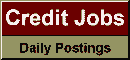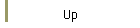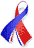DefaultRisk.com the web's biggest credit risk modeling resource.doi> search: A or B Cited by these papersRelated articlesAlternative sources Export citation to:- HTML - Text (plain)- BibTeX - RIS - ReDIF

Impact of the First to Default Time on Bilateral CVA

by Damiano Brigo of the King's College, London,
Cristin Buescu of the King's College, London, and
Massimo Morini of the Banca IMI & Bocconi University, Milan

June 20, 2011

Abstract: We compare two different bilateral counterparty valuation adjustment (BVA) formulas. The first formula is an approximation and is based on subtracting the two unilateral Credit Valuation Adjustment (CVA)'s formulas as seen from the two different parties in the transaction. This formula is only a simplified representation of bilateral risk and ignores that upon the first default closeout proceedings are ignited. As such, it involves double counting. We compare this formula with the fully specified bilateral risk formula, where the first to default time is taken into account. The latter correct formula depends on default dependence between the two parties, whereas the simplified one does not. We also analyze a candidate simplified formula in case the replacement closeout is used upon default, following ISDA's recommendations, and we find the simplified formula to be the same as in the risk free closeout case. We analyze the error that is encountered when using the simplified formula in a couple of simple products: a zero coupon bond, where the exposure is unidirectional, and an equity forward contract where exposure can go both ways. For the latter case we adopt a bivariate exponential distribution due to Gumbel  to model the joint default risk of the two parties in the deal. We present a number of realistic cases where the simplified formula differs considerably from the correct one.

Keywords: Credit Valuation Adjustment, Unilateral CVA, Bilateral CVA, Simplified Bilateral CVA, Debit Valuation Adjustment, Closeout, Equity Forward Contract, Zero coupon bond, Bivariate exponential distributions, Gumbel bivariate exponential distributions

Books Referenced in this paper:  (what is this?)